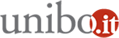# Statistics seminar 2018 - On Wong-Zakai approximation theorems for Ito stochastic differential equations

24/01/2018 dalle 14:30

Dove Dipartimento di Scienze Statistiche - Via Belle Arti 41 - Aula SeminariAggiungi l'evento al calendario

Relatore
Alberto Lanconelli
Università di Bari

Abstract
From a modeling point of view the celebrated Wong-Zakai theorem provides a crucial insight in the theory of stochastic differential equations. It asserts that the solution to certain random differential equations, which can be considered as natural approximations to stochastic differential equations, converges to the solution of the Stratonovich interpretation of the SDE, instead of the more popular Ito's one. The reason for that has to be found in the type of product utilized in the random differential equation to multiply the diffusion coefficient with the smooothed white noise. In this talk we will suggest how the aformentioned random differential equation can be modified in order to obtain the Ito interpretation in the limit. This will be done using the notion of Wick product, which is to some extent the adjoint of the Malliavin derivative operator. The issue on the speed of convergence will also be addressed.

Organizzazione
Enrico Bernardi